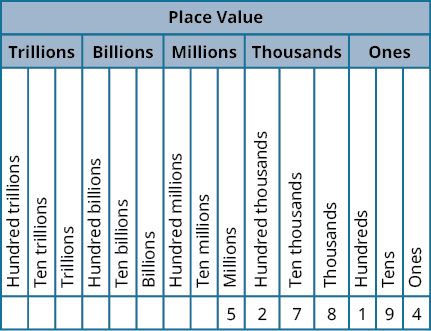## Key Concepts• Name a whole number in words.
1. Starting at the digit on the left, name the number in each period, followed by the period name. Do not include the period name for the ones.
2. Use commas in the number to separate the periods.
• Use place value to write a whole number.
1. Identify the words that indicate periods. (Remember the ones period is never named.)
2. Draw three blanks to indicate the number of places needed in each period.
3. Name the number in each period and place the digits in the correct place value position.
• Round a whole number to a specific place value.
1. Locate the given place value. All digits to the left of that place value do not change.
2. Underline the digit to the right of the given place value.
3. Determine if this digit is greater than or equal to 5. If yes—add 1 to the digit in the given place value. If no—do not change the digit in the given place value.
• Replace all digits to the right of the given place value with zeros.

## Glossary

coordinate
A number paired with a point on a number line is called the coordinate of the point.
counting numbers or natural numbers
The counting numbers are the numbers 1, 2, 3, ….
number line
A number line is used to visualize numbers. The numbers on the number line get larger as they go from left to right, and smaller as they go from right to left.
origin
The origin is the point labeled 0 on a number line.
place value system
Our number system is called a place value system because the value of a digit depends on its position, or place, in a number.
rounding
The process of approximating a number is called rounding.
whole numbers
The whole numbers are the numbers 0, 1, 2, 3, ….